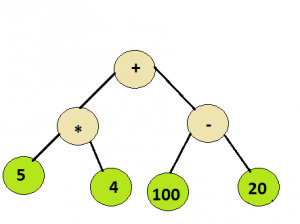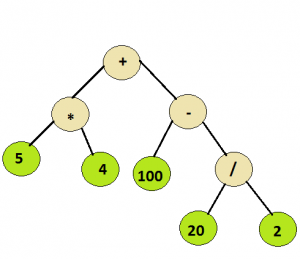Related Articles

# Evaluation of Expression Tree

• Difficulty Level : Medium
• Last Updated : 13 Apr, 2021

Given a simple expression tree, consisting of basic binary operators i.e., + , – ,* and / and some integers, evaluate the expression tree.

Examples:

```
Input :
Root node of the below treeOutput :
100

Input :
Root node of the below treeOutput :
110
```

## Recommended: Please solve it on “PRACTICE” first, before moving on to the solution.

As all the operators in the tree are binary hence each node will have either 0 or 2 children. As it can be inferred from the examples above , the integer values would appear at the leaf nodes , while the interior nodes represent the operators.
To evaluate the syntax tree , a recursive approach can be followed .

```Algorithm :
Let t be the syntax tree
If  t is not null then
If t.info is operand then
Return  t.info
Else
A = solve(t.left)
B = solve(t.right)
return A operator B
where operator is the info contained in t

```

The time complexity would be O(n), as each node is visited once. Below is a C++ program for the same:

## C/C++

 `// C++ program to evaluate an expression tree ``#include  ``using` `namespace` `std; `` ` `// Class to represent the nodes of syntax tree ``class` `node ``{ ``public``: ``    ``string info; ``    ``node *left = NULL, *right = NULL; ``    ``node(string x) ``    ``{ ``        ``info = x; ``    ``} ``}; `` ` `// Utility function to return the integer value ``// of a given string ``int` `toInt(string s) ``{ ``    ``int` `num = 0; ``       ` `      ``// Check if the integral value is ``      ``// negative or not``      ``// If it is not negative, generate the number ``      ``// normally``      ``if``(s!=``'-'``)``        ``for` `(``int` `i=0; ileft && !root->right) ``        ``return` `toInt(root->info); `` ` `    ``// Evaluate left subtree ``    ``int` `l_val = eval(root->left); `` ` `    ``// Evaluate right subtree ``    ``int` `r_val = eval(root->right); `` ` `    ``// Check which operator to apply ``    ``if` `(root->info==``"+"``) ``        ``return` `l_val+r_val; `` ` `    ``if` `(root->info==``"-"``) ``        ``return` `l_val-r_val; `` ` `    ``if` `(root->info==``"*"``) ``        ``return` `l_val*r_val; `` ` `    ``return` `l_val/r_val; ``} `` ` `//driver function to check the above program ``int` `main() ``{ ``    ``// create a syntax tree ``    ``node *root = ``new` `node(``"+"``); ``    ``root->left = ``new` `node(``"*"``); ``    ``root->left->left = ``new` `node(``"5"``); ``    ``root->left->right = ``new` `node(``"-4"``); ``    ``root->right = ``new` `node(``"-"``); ``    ``root->right->left = ``new` `node(``"100"``); ``    ``root->right->right = ``new` `node(``"20"``); ``    ``cout << eval(root) << endl; `` ` `    ``delete``(root); `` ` `    ``root = ``new` `node(``"+"``); ``    ``root->left = ``new` `node(``"*"``); ``    ``root->left->left = ``new` `node(``"5"``); ``    ``root->left->right = ``new` `node(``"4"``); ``    ``root->right = ``new` `node(``"-"``); ``    ``root->right->left = ``new` `node(``"100"``); ``    ``root->right->right = ``new` `node(``"/"``); ``    ``root->right->right->left = ``new` `node(``"20"``); ``    ``root->right->right->right = ``new` `node(``"2"``); `` ` `    ``cout << eval(root); ``    ``return` `0; ``} `

## Python

 `# Python program to evaluate expression tree`` ` `# Class to represent the nodes of syntax tree``class` `node:``    ``def` `__init__(``self``, value):``        ``self``.left ``=` `None``        ``self``.data ``=` `value``        ``self``.right ``=` `None`` ` `# This function receives a node of the syntax tree``# and recursively evaluate it``def` `evaluateExpressionTree(root):`` ` `    ``# empty tree``    ``if` `root ``is` `None``:``        ``return` `0`` ` `    ``# leaf node``    ``if` `root.left ``is` `None` `and` `root.right ``is` `None``:``        ``return` `int``(root.data)`` ` `    ``# evaluate left tree``    ``left_sum ``=` `evaluateExpressionTree(root.left)`` ` `    ``# evaluate right tree``    ``right_sum ``=` `evaluateExpressionTree(root.right)`` ` `    ``# check which operation to apply``    ``if` `root.data ``=``=` `'+'``:``        ``return` `left_sum ``+` `right_sum``     ` `    ``elif` `root.data ``=``=` `'-'``:``        ``return` `left_sum ``-` `right_sum``     ` `    ``elif` `root.data ``=``=` `'*'``:``        ``return` `left_sum ``*` `right_sum``     ` `    ``else``:``        ``return` `left_sum ``/` `right_sum`` ` `# Driver function to test above problem``if` `__name__``=``=``'__main__'``:``     ` `    ``# creating a sample tree``    ``root ``=` `node(``'+'``)``    ``root.left ``=` `node(``'*'``)``    ``root.left.left ``=` `node(``'5'``)``    ``root.left.right ``=` `node(``'4'``)``    ``root.right ``=` `node(``'-'``)``    ``root.right.left ``=` `node(``'100'``)``    ``root.right.right ``=` `node(``'20'``)``    ``print` `evaluateExpressionTree(root)`` ` `    ``root ``=` `None`` ` `    ``#creating a sample tree``    ``root ``=` `node(``'+'``)``    ``root.left ``=` `node(``'*'``)``    ``root.left.left ``=` `node(``'5'``)``    ``root.left.right ``=` `node(``'4'``)``    ``root.right ``=` `node(``'-'``)``    ``root.right.left ``=` `node(``'100'``)``    ``root.right.right ``=` `node(``'/'``)``    ``root.right.right.left ``=` `node(``'20'``)``    ``root.right.right.right ``=` `node(``'2'``)``    ``print` `evaluateExpressionTree(root)`` ` `# This code is contributed by Harshit Sidhwa`

Output:
```60
110
```

This article is contributed by Ashutosh Kumar. If you like GeeksforGeeks and would like to contribute, you can also write an article using contribute.geeksforgeeks.org or mail your article to contribute@geeksforgeeks.org. See your article appearing on the GeeksforGeeks main page and help other Geeks.